# Simple Curves and SurfacesWe have seen the simplest curves (lines) and surfaces (planes) in the previous page. Next to lines and planes, there are conics and quadric surfaces. Although conics and quadric surfaces have been around for about 2000 years, they are still the most popular objects in many computer aided design and modeling systems. We shall discuss conics, quadric surfaces and tori on this page only. Consulting your calculus and/or geometry books should be very helpful. Your linear algebra book should also cover some of these topics with a modern approach.

The following figures show to you three different ways of cutting a cone with a plane. The conic sections, from left to right, are an ellipse, a hyperbola and a parabola.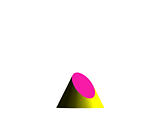## Curves

### Circles

The simplest non-linear curve is unquestionably the circle. A circle with center (a,b) and radius r has an equation as follows:
(x - a)2 + (x - b)2 = r2
If the center is the origin, the above equation is simplified to
x2 + y2 = r2
The above equations are referred to as the implicit form of the circle. The parametric form of a circle is
x = rcos(t)
y = rsin(t)
The following is the parametric form of a circle whose center is not the origin:
x = a + rcos(t)
y = b + rsin(t)
The above parametric form uses trigonometric functions. We shall discuss a parametric form of a circle without trigonometric functions later.

### Conics in Normal Forms

A direct generalization of the circle is the so-called conic curves or simply conics. Greeks knew about conics very well. In fact, Apollonius of Perga (262 - 200 B.C.) wrote a book of several volumes about conics. Conics are the intersection curves of a plane and a circular cone (i.e., a cone whose base is a circle and whose axis is perpendicular to the base and through the center of the base circle).

There are three types of non-degenerate conics: ellipses, hyperbolas and parabolas. Ellipses and hyperbolas are called central conics because they have a center of symmetry, while parabolas are non-central.

The normal form of an ellipse is the following implicit equation: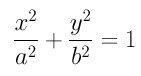The axes of this ellipse are the x- and y-axis, a and b are the axis lengths, and the larger one of a and b is the major axis while the smaller one is the minor axis. It is not difficult to see that an ellipse in this form has the following parametric form:

x = acos(t)
y = bsin(t)

The normal form of a hyperbola is the following implicit equation: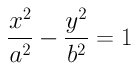The definition of major axis and minor axis are identical to that of ellipses. The x-axis intersects the curve at two points (a, 0) and (-a, 0) and, the y-axis does not intersect the curve at all. A possible parametric form of the above hyperbola is the following:

x = asec(t)
y = btan(t)

The center of an ellipse and hyperbola, in normal form, is the coordinate origin and the curve is symmetric about its center and its axes.

The normal form of a parabola is the following implicit equation: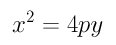In this normal form, for any point (x,y) on a parabola, the value of y must be positive and the opening of this parabola is upward. The axis of this parabola is the y-axis. It is intersecting to note that the normal form of a parabola is already a parametric form. Or, if you like, you can rewrite it into the following:

x = t
y = t2 / (4p)

### Conics in General Form

Conics are degree two curves because their most general form is the following degree two implicit polynomial: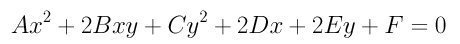In the above polynomial, the coefficients of xy, x and y are 2B, 2D and 2E, respectively. This polynomial has six coefficients; however, dividing it with a non-zero coefficient would reduce six to five. Thus, in general, five conditions can uniquely determine a conic. In linear algebra, you perhaps have learned the way of reducing the above polynomial to a normal form using eigenvalues and eigenvectors.

Frequently, we only want to know the curve type of a general second degree polynomial. In this case, as long as the second degree equation represents a conic rather than two intersecting or parallel lines, it can easily be done as follows:

1. If B2 < A*C, the general equation represents an ellipse.
2. IF B2 = A*C, the general equation represents a parabola.
3. If B2 > A*C, the general equation represents a hyperbola.
Expression B2-A*C is called the discriminant of the general second degree polynomial. Based on the above, if the value of the discriminant is less than, equal to or greater than zero, the conic is an ellipse, a parabola, or a hyperbola.

### Conics in Matrix Form

One nice thing of conics is that its general form can be rewritten compactly using matrices. First, each point x = (x, y) is considered as a column vector whose third component is 1 and hence the transpose is a row vector, written as xT = [ x, y, 1 ]. Next, the six coefficients of the general second degree polynomial are used to construct a three-by-three symmetric matrix as follows: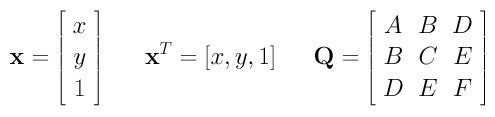It is not difficult to verify that the general second degree polynomial becomes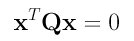Now what you have learned from linear algebra can be applied to this matrix form.## Surfaces

### Quadric Surfaces in Normal Forms

Quadric surfaces, or quadrics for short, consist of the following different types: ellipsoids, hyperboloids of one sheet, hyperboloids of two sheets, elliptic paraboloids, and hyperboloid paraboloids. The following are their normal forms in implicit forms and their shapes:

 Ellipsoid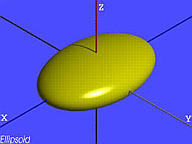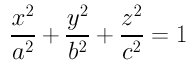Hyperboloid of One Sheet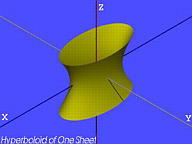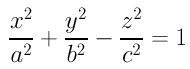Hyperboloid of Two Sheets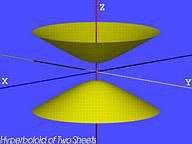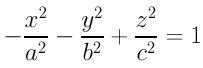Elliptic Paraboloid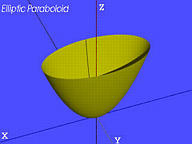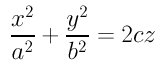Hyperbolic Paraboloid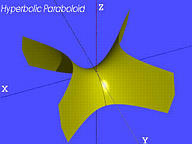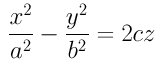These five quadric surfaces are normally referred to as rank four quadrics. There are two types of rank three quadrics: cones and cylinders. Cylinders have three subtypes: elliptic cylinders, hyperbolic cylinders and parabolic cylinders as shown below:

 Cone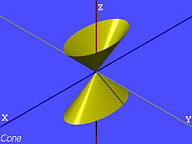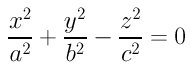Elliptic Cylinder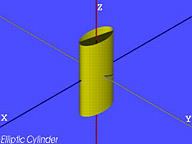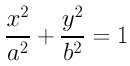Hyperbolic Cylinder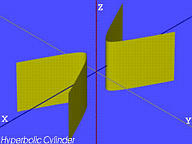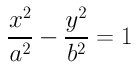Parabolic Cylinder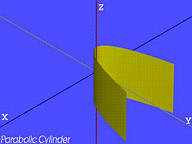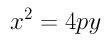### Quadric Surfaces in General Form

The general form of quadric surfaces is the following: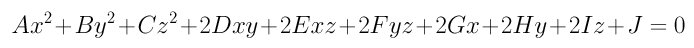It has ten coefficients; but, as mentioned in the discussion of conics, dividing the equation with one of its non-zero coefficients reduces the number of coefficients to nine. Please also note that except for the coefficients for x2, y2 and z2 and the constant term, all coefficients has a multiplier of 2.

You might want to ask a question: is it possible to develop an algorithm for classifying the quadrics just like we did for conics? More precisely, if a general second degree polynomial is given, could we tell its type (i.e., ellipsoid, hyperboloid of one sheet, elliptic paraboloid, etc) by simply looking at their coefficients? The answer is always a "yes"; but the computation algorithm is quite complex. So, I would rather skip this algorithm. However, you can always use eigenvalues and eigenvectors to solve this problem.

### Quadric Surfaces in Matrix Form

The equation of a general quadric can also be put into matrix form: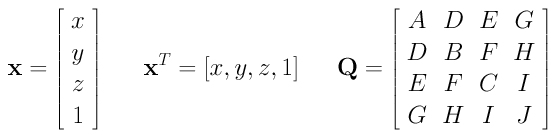where (x, y, z) is the coordinates of a point. This form translates the general second polynomial of a quadric to the following matrix form:Note that it is exactly identical to that of a conic. Therefore, matrices help to bring conics and quadrics into an identical form.

After knowing the matrix form of quadrics, we will be able to discuss the meaning of rank four and rank three quadrics. Consider the symmetric matrix Q that contains the coefficients of a general second degree polynomial. The rank of a matrix is the number of non-zero eigenvalues. Thus, rank four quadrics are those quadrics whose matrix Q are of rank four. It is easy to see (from their normal forms) that ellipsoids, hyperboloids and paraboloids are rank four quadrics, and cones and cylinders are rank three quadrics. If a general second degree polynomial factors into the product of two distinct degree one polynomials (i.e., planes), Q will have rank two.

### Tori in Normal Form

A torus can be generated by rotating a circle, the minor circle, about a line, the rotation axis or axis of rotation. The moving circle has its center on another circle, the major circle. The radii of the major and minor circles are referred to as the major radius and minor radius, denoted by R and r, respectively.

If the rotation axis is the z-axis and the major circle lies on the xy-plane, the equation of the generated torus is the following: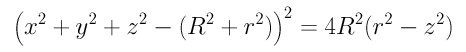If R is greater than r, the result is a commonly seen torus as shown in the middle of the following figure.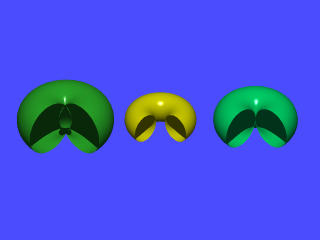IF R is equal to r, then all moving circles are tangent to the rotation axis at the coordinate origin as shown in the right figure. If R is less than r, all moving circles intersect the rotation axis at two distinct points and the generated torus will have a olive like shape in the interior of the torus as shown in the left figure.